# RD Sharma Class 8 Solutions Chapter 27 Introduction to Graphs Ex 27.2

In this chapter, we provide RD Sharma Class 8 Solutions Chapter 27 Introduction to Graphs Ex 27.2 for English medium students, Which will very helpful for every student in their exams. Students can download the latest RD Sharma Class 8 Solutions Chapter 27 Introduction to Graphs Ex 27.2 pdf, free RD Sharma Class 8 Solutions Chapter 27 Introduction to Graphs Ex 27.2 book pdf download. Now you will get step by step solution to each question.

### RD Sharma Class 8 Solutions Chapter 27 Introduction to Graphs Ex 27.2Download PDF

Question 1.
The following table shows the number of patients discharged from a hospital with HIV diagnosis in different years :Represent this information by a graph.
Solution:
Represent years along x-axis and number of patients along y-axis. Now, plot the points (2002, 150), (2003, 170), (2004, 195), (2005, 225) and (2006, 230) on the graph and join them in order. We get the required graph as shown on the graph.Question 2.
The following table shows the amount of rice grown by a farmer in different years :Plot a graph to illustrate this information.
Solution:
We represent years along x-axis and rice (in quintals) along y-axis. Now we plot the points (2000,200), (2001, 180), (2002,240), (2003,260), (2004,250), (2005,200) and (2006,270) on the graph and join them in order as shown on the graph.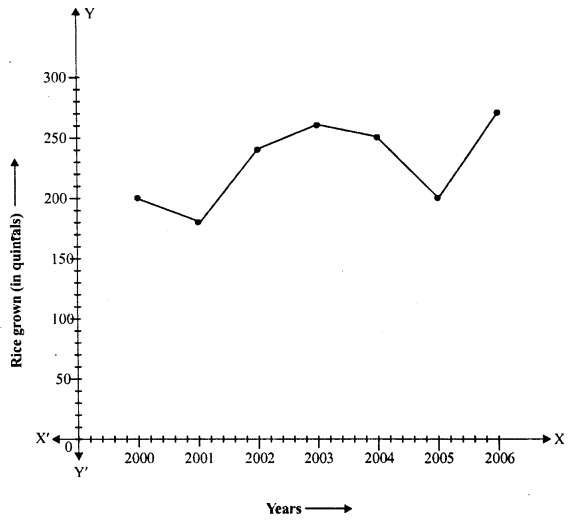Question 3.
The following table gives the information regarding the number of persons employed to a piece of work and time taken to complete the work:Plot a graph of this information.
Solution:
We represent number of person along x-axis and time taken in day along y-axis. Now plot the points (2,12), (4,6), (6,4) and (8, 3) on the graph and join them in order as shown on the graph.Question 4.
The following table gives the information regarding length of a side of a square and its area :Draw a graph to illustrate this information.
Solution:
We represent length of a side (in cm) along x-axis and Area of square (in cm2) along the y-axis. Now plot the points (1,1), (2, 4), (3, 9), (4, 16) and (5, 25) on the graph and join them in order. We get the required graph as shown on the graph.Question 5.
The following table shows the sales of a commodity during the years 2000 to 2006.Draw a graph of this information.
Solution:
We represent years on x-axis and sales (in lakh rupees) along y-axis. Now plot the points
(2000, 1.5), (2001, 1.8), (2002, 2.4), (2003, 3.2), (2004, 5.4), (2005, 7.8) and (2006, 8.6) on the graph and join them in order we get the required graph as shown.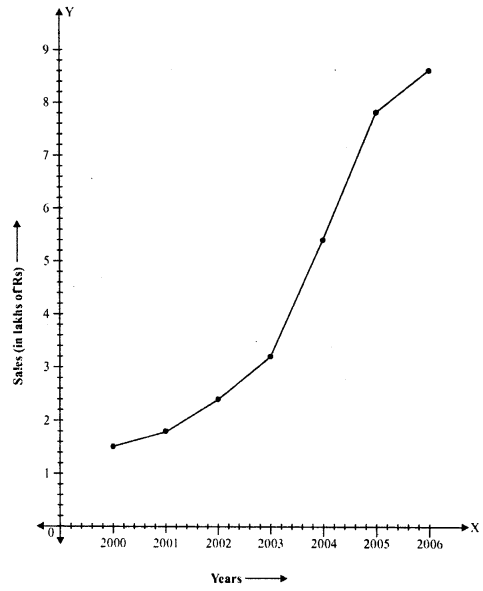Question 6.
Draw the temperature-time graph in each of the following cases :Solution:
(i) We represent time along x-axis and temperature (in °F) alongy-axis. Now plot the points (7:00, 100), (9:00, 101), (11:00, 104), (13:00, 102), (15:00, 100), (17:00, 99), (19:00, 100) and (21:00, 98) on the graph and join them in order to get the required graph as shown on the graph.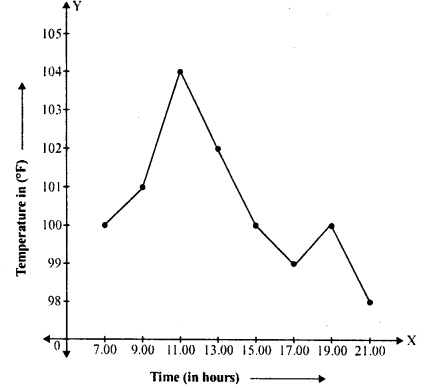(ii) We represent time along x-axis and temperature (in °F) along j-axis. Now plot the points (8:00, 100), (i0:00, 101), (12:00, 104), (14:00, 103), (16:00, 99), (18:00,98), (20:00,100) on the graph and join them in order to get the required graph as shown on the graph.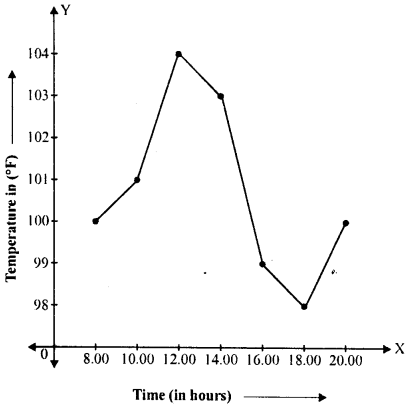Question 7.
Draw the velocity-time graph from the following data :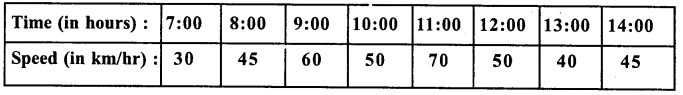Solution:
We represent time (in hours) along x- axis and speed (in km/hr) along y-axis. Now plot the points (7:00,30), (8:00,45), (9:00,60), (10:00, 50), (11:00, 70), (12:00, 50), (13:00, 40) and (14:00,45) on the graph and join them in order to get the required graph as shown.Question 8.
The runs scored by a cricket team in first 15 overs are given below :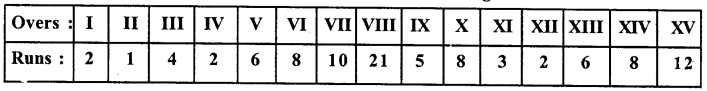Draw the graph representing the above data in two different ways as a graph and as a bar chart.
Solution:
We represent overs along.t-axis and runs along v-axis. Now plot the points (1,2), (II, 1), (III, 4), (IV, 2), (V, 6), (VI, 8), (VII, 10), (VIII, 21), (IX, 5), (X, 8), (XI, 3), (XII, 2), (XIII, 6), (XIV, 8), (XV, 12) on the graph and join them to get the graph, as shown bar graph of the given data is given below:Question 9.
The runs scored by two teams A and B in first 10 overs are given below :Draw a graph depicting the data, making the graphs on the same axes in each case in two different ways as a graph and as a bar chart.
Solution:
We represent overs along x-axis and runs scored by team A and team B with different types of lines along y-axis.
Plot the points for team A : (I, 2), (II, 1), (III,8), (IV, 9), (V, 4), (VI, 5), (VII, 6), (VIII, 10), (IX, 6) and (X, 2)
and for team B, the points will be : (I, 5), (II, 6), (III, 2), (IV, 10), (V, 5), (VI, 6), (VII, 3), (VIII, 4), (IX, 8), (X, 10).
Then join them in order to get the required graph for team A and team B as shown.
Note : For team A ………
for team B …………All Chapter RD Sharma Solutions For Class 8 Maths

—————————————————————————–

All Subject NCERT Exemplar Problems Solutions For Class 8

All Subject NCERT Solutions For Class 8

*************************************************

I think you got complete solutions for this chapter. If You have any queries regarding this chapter, please comment on the below section our subject teacher will answer you. We tried our best to give complete solutions so you got good marks in your exam.

If these solutions have helped you, you can also share rdsharmasolutions.in to your friends.